JMSLTM Numerical Library 7.2.0
com.imsl.math

Class ComplexSuperLU

• All Implemented Interfaces:
Serializable

public class ComplexSuperLU
extends Object
implements Serializable
Computes the LU factorization of a general sparse matrix of type ComplexSparseMatrix by a column method and solves a sparse linear system of equations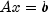.

Consider the sparse linear system of equations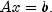Here, A is a general square, nonsingular, n by n sparse matrix, and x and b are vectors of length n. All entries in A, x and b are of type Complex.

Gaussian elimination, applied to the system above, can be shortly described as follows:

1. Compute a triangular factorization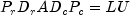. Here,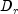and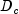are positive definite diagonal matrices to equilibrate the system and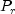and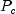are permutation matrices to ensure numerical stability and preserve sparsity. L is a unit lower triangular matrix and U is an upper triangular matrix.
2. Solveby evaluating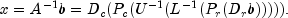This is done efficiently by multiplying from right to left in the last expression: Scale the rows of b by. Multiplying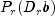means permuting the rows of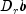. Multiplying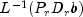means solving the triangular system of equations with matrix L by substitution. Similarly, multiplying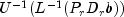means solving the triangular system with U.

Class ComplexSuperLU handles step 1 above in the solve method if it is has not been computed prior to step 2. More precisely, beforeis solved the following steps are performed:

1. Equilibrate matrix A, i.e. compute diagonal matricesandso that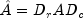is "better conditioned" than A, i.e.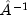is less sensitive to perturbations in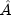than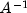is to perturbations in A.
2. Order the columns ofto increase the sparsity of the computed L and U factors, i.e. replaceby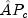whereis a column permutation matrix.
3. Compute the LU factorization of. For numerical stability, the rows ofare eventually permuted through the factorization process by scaled partial pivoting, leading to the decomposition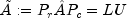. The LU factorization is done by a left looking supernode-panel algorithm with 2-D blocking. See Demmel, Eisenstat, Gilbert et al. (1999) for further information on this technique.
4. Compute the reciprocal pivot growth factor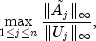where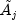and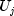denote the j-th column of matrices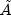and U, respectively.
5. Estimate the reciprocal of the condition number of matrix.

Method solve uses this information to perform the following steps:

1. Solve the systemusing the computed triangular factors.
2. Iteratively refine the solution, again using the computed triangular factors. This is equivalent to Newton's method.
3. Compute forward and backward error bounds for the solution vector x.

Some of the steps mentioned above are optional. Their settings can be controlled by the set methods of class ComplexSuperLU.

Class ComplexSuperLU is based on the SuperLU code written by Demmel, Gilbert, Li et al. For more detailed explanations of the factorization and solve steps, see the SuperLU Users' Guide (1999).

Copyright (c) 2003, The Regents of the University of California, through Lawrence Berkeley National Laboratory (subject to receipt of any required approvals from U.S. Dept. of Energy)

Redistribution and use in source and binary forms, with or without modification, are permitted provided that the following conditions are met:

(1) Redistributions of source code must retain the above copyright notice, this list of conditions and the following disclaimer.

(2) Redistributions in binary form must reproduce the above copyright notice, this list of conditions and the following disclaimer in the documentation and/or other materials provided with the distribution.

(3) Neither the name of Lawrence Berkeley National Laboratory, U.S. Dept. of Energy nor the names of its contributors may be used to endorse or promote products derived from this software without specific prior written permission.

THIS SOFTWARE IS PROVIDED BY THE COPYRIGHT HOLDERS AND CONTRIBUTORS "AS IS" AND ANY EXPRESS OR IMPLIED WARRANTIES, INCLUDING, BUT NOT LIMITED TO, THE IMPLIED WARRANTIES OF MERCHANTABILITY AND FITNESS FOR A PARTICULAR PURPOSE ARE DISCLAIMED. IN NO EVENT SHALL THE COPYRIGHT OWNER OR CONTRIBUTORS BE LIABLE FOR ANY DIRECT, INDIRECT, INCIDENTAL, SPECIAL, EXEMPLARY, OR CONSEQUENTIAL DAMAGES (INCLUDING, BUT NOT LIMITED TO, PROCUREMENT OF SUBSTITUTE GOODS OR SERVICES; LOSS OF USE, DATA, OR PROFITS; OR BUSINESS INTERRUPTION) HOWEVER CAUSED AND ON ANY THEORY OF LIABILITY, WHETHER IN CONTRACT, STRICT LIABILITY, OR TORT (INCLUDING NEGLIGENCE OR OTHERWISE) ARISING IN ANY WAY OUT OF THE USE OF THIS SOFTWARE, EVEN IF ADVISED OF THE POSSIBILITY OF SUCH DAMAGE.

Example, Serialized Form
• Field Summary

Fields
Modifier and Type Field and Description
static int COLUMN_APPROXIMATE_MINIMUM_DEGREE
For column ordering, use column approximate minimum degree ordering.
static int COLUMN_SCALING
Indicates that input matrix A was column scaled before factorization.
static int FILL_FACTOR
A performance tuning parameter which can be adjusted via method setPerformanceTuningParameters.
static int MAXIMUM_SUPERNODE_SIZE
A performance tuning parameter which can be adjusted via method setPerformanceTuningParameters.
static int MINIMUM_COLUMN_DIMENSION
A performance tuning parameter which can be adjusted via method setPerformanceTuningParameters.
static int MINIMUM_DEGREE_AT_A
For column ordering, use minimum degree ordering on the structure of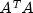.
static int MINIMUM_DEGREE_AT_PLUS_A
For column ordering, use minimum degree ordering on the structure of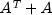.
static int MINIMUM_ROW_DIMENSION
A performance tuning parameter which can be adjusted via method setPerformanceTuningParameters.
static int NATURAL_ORDERING
For column ordering, use the natural ordering.
static int NO_SCALING
Indicates that input matrix A was not equilibrated before factorization.
static int PANEL_SIZE
A performance tuning parameter which can be adjusted via method setPerformanceTuningParameters.
static int RELAXATION_PARAMETER
A performance tuning parameter which can be adjusted via method setPerformanceTuningParameters.
static int ROW_AND_COLUMN_SCALING
Indicates that input matrix A was row and column scaled before factorization.
static int ROW_SCALING
Indicates that input matrix A was row scaled before factorization.
• Field Detail

• COLUMN_APPROXIMATE_MINIMUM_DEGREE

public static final int COLUMN_APPROXIMATE_MINIMUM_DEGREE
For column ordering, use column approximate minimum degree ordering.
Constant Field Values
• COLUMN_SCALING

public static final int COLUMN_SCALING
Indicates that input matrix A was column scaled before factorization. This is a return value for getEquilibrationMethod.
Constant Field Values
• FILL_FACTOR

public static final int FILL_FACTOR
A performance tuning parameter which can be adjusted via method setPerformanceTuningParameters.
Constant Field Values
• MAXIMUM_SUPERNODE_SIZE

public static final int MAXIMUM_SUPERNODE_SIZE
A performance tuning parameter which can be adjusted via method setPerformanceTuningParameters.
Constant Field Values
• MINIMUM_COLUMN_DIMENSION

public static final int MINIMUM_COLUMN_DIMENSION
A performance tuning parameter which can be adjusted via method setPerformanceTuningParameters.
Constant Field Values
• MINIMUM_DEGREE_AT_A

public static final int MINIMUM_DEGREE_AT_A
For column ordering, use minimum degree ordering on the structure of.
Constant Field Values
• MINIMUM_DEGREE_AT_PLUS_A

public static final int MINIMUM_DEGREE_AT_PLUS_A
For column ordering, use minimum degree ordering on the structure of.
Constant Field Values
• MINIMUM_ROW_DIMENSION

public static final int MINIMUM_ROW_DIMENSION
A performance tuning parameter which can be adjusted via method setPerformanceTuningParameters.
Constant Field Values
• NATURAL_ORDERING

public static final int NATURAL_ORDERING
For column ordering, use the natural ordering.
Constant Field Values
• NO_SCALING

public static final int NO_SCALING
Indicates that input matrix A was not equilibrated before factorization. This is a return value for getEquilibrationMethod.
Constant Field Values
• PANEL_SIZE

public static final int PANEL_SIZE
A performance tuning parameter which can be adjusted via method setPerformanceTuningParameters.
Constant Field Values
• RELAXATION_PARAMETER

public static final int RELAXATION_PARAMETER
A performance tuning parameter which can be adjusted via method setPerformanceTuningParameters.
Constant Field Values
• ROW_AND_COLUMN_SCALING

public static final int ROW_AND_COLUMN_SCALING
Indicates that input matrix A was row and column scaled before factorization. This is a return value for getEquilibrationMethod.
Constant Field Values
• ROW_SCALING

public static final int ROW_SCALING
Indicates that input matrix A was row scaled before factorization. This is a return value for getEquilibrationMethod.
Constant Field Values
• Constructor Detail

• ComplexSuperLU

public ComplexSuperLU(ComplexSparseMatrix A)
Constructor for ComplexSuperLU.
Parameters:
A - a ComplexSparseMatrix containing the sparse quadratic input matrix.
• Method Detail

• getColumnPermutationMethod

public int getColumnPermutationMethod()
Returns the method that will be used to permute the columns of the input matrix.
Returns:
an int scalar specifying how the columns of the input matrix are to be permuted for sparsity preservation.
return value method
0 = NATURAL_ORDERING natural ordering, that is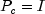, I the identity matrix
1 = MINIMUM_DEGREE_AT_PLUS_A minimum degree ordering on the structure of2 = MINIMUM_DEGREE_AT_A minimum degree ordering on the structure of3 = COLUMN_APPROXIMATE_MINIMUM_DEGREE column approximate minimum degree ordering
• getConditionNumber

public double getConditionNumber()
throws SingularMatrixException
Returns the estimate of the reciprocal condition number of the matrix A.
Returns:
a double scalar containing the reciprocal condition number of the matrix A after equilibration and permutation of rows/columns (if done). If the reciprocal condition number is less than machine precision, in particular if it is equal to 0, the matrix is singular to working precision.
Throws:
SingularMatrixException
• getDiagonalPivotThreshold

public double getDiagonalPivotThreshold()
Returns the threshold used for a diagonal entry to be an acceptable pivot.
Returns:
a double scalar specifying the threshold used for a diagonal entry to be an acceptable pivot.
• getEquilibrate

public boolean getEquilibrate()
Returns the equilibration flag.
Returns:
a boolean specifying whether or not matrix A is equilibrated before factorization. If getEquilibrate returns true the system is equilibrated, if getEquilibrate returns false, no equilibration is performed.
• getEquilibrationMethod

public int getEquilibrationMethod()
Returns information on the type of equilibration used before matrix factorization.
Returns:
an int value specifying the equilibration option used.
return value option description
1 = NO_SCALING No equilibration is performed.
2 = ROW_SCALING Equilibration is performed with row scaling.
3 = COLUMN_SCALING Equilibration is performed with column scaling.
4 = ROW_AND_COLUMN_SCALING Equilibration is performed with row and column scaling.
• getForwardErrorBound

public double getForwardErrorBound()
Returns the estimated forward error bound for each solution vector.
Returns:
a double containing the estimated forward error bound for the solution vector. The estimate is as reliable as the estimate for the reciprocal condition number, and is almost always a slight overestimate of the true error. If iterative refinement is not used, the return value = 1.0.
• getIterativeRefinement

public boolean getIterativeRefinement()
Returns a value specifying whether iterative refinement is to be performed or not.
Returns:
a boolean scalar specifying whether iterative refinement is to be performed, true, or no iterative refinement is to be performed, false.
• getPerformanceTuningParameters

public int getPerformanceTuningParameters(int parameter)
Returns a performance tuning parameter value.
Parameters:
parameter - an int scalar that specifies the parameter for which the value is to be returned.
parameter return value description
PANEL_SIZE The panel size.
RELAXATION_PARAMETER The relaxation parameter to control supernode amalgamation.
MAXIMUM_SUPERNODE_SIZE The maximum allowable size for a supernode.
MINIMUM_ROW_DIMENSION The minimum row dimension to be used for 2D blocking.
MINIMUM_COLUMN_DIMENSION The minimum column dimension to be used for 2D blocking.
FILL_FACTOR The estimated fill factor for L and U, compared with A.

Returns:
an int specifying the current value used for the specified tuning parameter.
• getPivotGrowth

public boolean getPivotGrowth()
Returns the reciprocal pivot growth factor flag.
Returns:
a boolean specifying whether to compute the reciprocal pivot growth factor. Returns true if the reciprocal pivot growth factor is to be computed.
• getReciprocalPivotGrowthFactor

public double getReciprocalPivotGrowthFactor()
throws SingularMatrixException
Returns the reciprocal pivot growth factor.
Returns:
a double scalar representing the reciprocal growth factor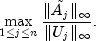If the returned value is much less than 1, the stability of the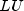factorization could be poor.
Throws:
SingularMatrixException
• getRelativeBackwardError

public double getRelativeBackwardError()
Returns the componentwise relative backward error of the solution vector.
Returns:
a double containing the componentwise relative backward error of the solution vector x. If setIterativeRefinement is not set to true, then getRelativeBackwardError returns 1.0.
• getSymmetricMode

public boolean getSymmetricMode()
Returns the symmetric mode flag.
Returns:
a boolean scalar indicating if symmetric mode is to be used. Returns true if symmetric mode is to be used.
• setColumnPermutationMethod

public void setColumnPermutationMethod(int colpermute)
Specifies how to permute the columns of the input matrix.
Parameters:
colpermute - an int scalar specifying how to permute the columns of the input matrix for sparsity preservation.
colpermute method
NATURAL_ORDERING natural ordering, that is, I the identity matrix
MINIMUM_DEGREE_AT_PLUS_A minimum degree ordering on the structure ofMINIMUM_DEGREE_AT_A minimum degree ordering on the structure ofCOLUMN_APPROXIMATE_MINIMUM_DEGREE column approximate minimum degree ordering
Default: colpermute = SuperLU.COLUMN_APPROXIMATE_MINIMUM_DEGREE.
• setDiagonalPivotThreshold

public void setDiagonalPivotThreshold(double thresh)
Specifies the threshold used for a diagonal entry to be an acceptable pivot.
Parameters:
thresh - a double scalar specifying the threshold used for a diagonal entry to be an acceptable pivot.
Default: thresh=1.0, i.e. classical partial pivoting.
Throws:
IllegalArgumentException - is thrown if thresh is not in the interval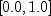.
• setEquilibrate

public void setEquilibrate(boolean equilibrate)
Specifies if input matrix A should be equilibrated before factorization.
Parameters:
equilibrate - a boolean determining if matrix A should be equilibrated before the factorization.

equilibrate action
false do not equilibrate
true equilibrate
Default: equilibrate = true.

• setIterativeRefinement

public void setIterativeRefinement(boolean refine)
Specifies whether to perform iterative refinement.
Parameters:
refine - a boolean scalar specifying whether to use iterative refinement, refine = true or no iterative refinement, refine = false.
Default: refine = false.
• setPerformanceTuningParameters

public void setPerformanceTuningParameters(int parameter,
int tunedValue)
Sets performance tuning parameters.
Parameters:
parameter - an int scalar that specifies the parameter to be tuned.
tunedValue - an int scalar that specifies the value to be used for the specified tuning parameter.
parameter Description Default
PANEL_SIZE The panel size. 10
RELAXATION_PARAMETER The relaxation parameter to control supernode amalgamation. 5
MAXIMUM_SUPERNODE_SIZE The maximum allowable size for a supernode. 100
MINIMUM_ROW_DIMENSION The minimum row dimension to be used for 2D blocking. 200
MINIMUM_COLUMN_DIMENSION The minimum column dimension to be used for 2D blocking. 40
FILL_FACTOR The estimated fill factor for L and U, compared with A. 20

Throws:
IllegalArgumentException - is thrown when a) parameter is not in the interval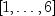or b) tunedValue is not greater than zero.
• setPivotGrowth

public void setPivotGrowth(boolean growth)
Specifies whether to compute the reciprocal pivot growth factor.
Parameters:
growth - a boolean specifying whether to compute the reciprocal pivot growth factor.
growth action
false don't compute growth factor
true compute growth factor
Default: growth = false.
• setSymmetricMode

public void setSymmetricMode(boolean symmetric)
Specifies whether to use the symmetric mode.
Parameters:
symmetric - a boolean indicating if symmetric mode is to be used. This mode should be applied if the input matrix A is diagonally dominant or nearly so. The user should then define a small diagonal pivot threshold (e.g. 0.0 or 0.01) by method setDiagonalPivotThreshold and choose an ()-based column permutation algorithm (e.g. column permutation method ComplexSuperLU.MINIMUM_DEGREE_AT_PLUS_A).
symmetric action
false symmetric mode is not used
true symmetric mode is used
Default: symmetric=false.
• solve

public Complex[] solve(Complex[] b)
throws SingularMatrixException
Computation of the solution vector for the system.
Parameters:
b - a Complex vector of length n, n the order of input matrix A, containing the right hand side.
Returns:
a Complex vector containing the solution to the system. Optionally, the solution is improved by iterative refinement, if setIterativeRefinement is set to true. Method solve internally first factorizes matrix A (step 1 of the introduction) if the factorization has not been done before.
Throws:
SingularMatrixException
• solveConjugateTranspose

public Complex[] solveConjugateTranspose(Complex[] b)
throws SingularMatrixException
Computation of the solution vector for the system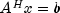.
Parameters:
b - a Complex vector of length n, n the order of input matrix A, containing the right hand side.
Returns:
a Complex vector containing the solution to the system. Optionally, the solution is improved by iterative refinement, if setIterativeRefinement is set to true . Method solveConjugateTranspose internally first factorizes matrix A (step 1 of the introduction) if the factorization has not been done before.
Throws:
SingularMatrixException
• solveTranspose

public Complex[] solveTranspose(Complex[] b)
throws SingularMatrixException
Computation of the solution vector for the system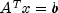.
Parameters:
b - a Complex vector of length n, n the order of input matrix A, containing the right hand side.
Returns:
a Complex vector containing the solution to the system. Optionally, the solution is improved by iterative refinement, if setIterativeRefinement is set to true . Method solveTranspose internally first factorizes matrix A (step 1 of the introduction) if the factorization has not been done before.
Throws:
SingularMatrixException
JMSLTM Numerical Library 7.2.0